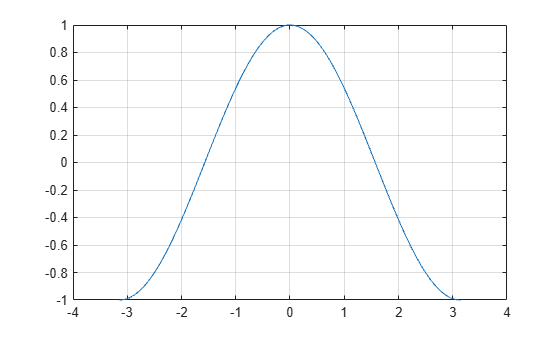Documentation

cos

Cosine of argument in radians

Description

Y = cos(X) returns the cosine for each element of X. The cos function operates element-wise on arrays. The function accepts both real and complex inputs.

• For real values of X, cos(X) returns real values in the interval [-1, 1].

• For complex values of X, cos(X) returns complex values.

Examples

collapse all

Plot the cosine function over the domain $-\pi \le x\le \pi$.

x = -pi:0.01:pi;
plot(x,cos(x))
grid onCalculate the cosine of the complex angles in vector x.

x = [-i pi+i*pi/2 -1+i*4];
y = cos(x)
y = 1×3 complex

1.5431 + 0.0000i  -2.5092 - 0.0000i  14.7547 +22.9637i

Input Arguments

collapse all

Input angle in radians, specified as a scalar, vector, matrix, or multidimensional array.

Data Types: single | double
Complex Number Support: Yes

Output Arguments

collapse all

Cosine of input angle, returned as a real-valued or complex-valued scalar, vector, matrix or multidimensional array.

collapse all

Cosine Function

The cosine of an angle, α, defined with reference to a right angled triangle isThe cosine of a complex argument, α, is

$\text{cos}\left(\alpha \right)=\frac{{e}^{i\alpha }+{e}^{-i\alpha }}{2}\text{\hspace{0.17em}}.$